## Example Questions

### Example Question #1 : Square Roots And Operations

Simplify: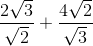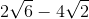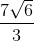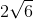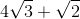Explanation:

Take each fraction separately first:

(2√3)/(√2) = [(2√3)/(√2)] * [(√2)/(√2)] = (2 * √3 * √2)/(√2 * √2) = (2 * √6)/2 = √6

Similarly:

(4√2)/(√3) = [(4√2)/(√3)] * [(√3)/(√3)] = (4√6)/3 = (4/3)√6

√6 + (4/3)√6 = (3/3)√6 + (4/3)√6 = (7/3)√6

### Example Question #37 : Arithmetic

Compare the quantities.

Quantity A: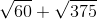Quantity B: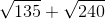Quantity B is larger.

Quantity A is larger.

The relationship cannot be determined from the information given.

The two quantities are equal.

The two quantities are equal.

Explanation:

Begin by breaking down each of the roots in question.  Often, for the GRE, your answer arises out of doing such basic "simplification moves".

Quantity AThis is the same as: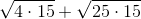, which can be reduced to: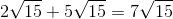Quantity BThis is the same as: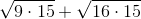, which can be reduced to: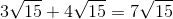Thus, at the end of working through the proper math, you realize that the two values are equal!

### Example Question #38 : Arithmetic

Simplify the following expression: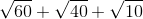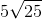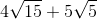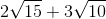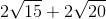Explanation:

Begin by factoring out each of the radicals: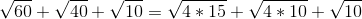For the first two radicals, you can factor out a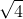or: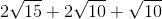The other root values cannot be simply broken down. Now, combine the factors with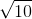: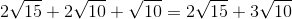### Example Question #1 : Square Roots And Operations

Solve for.

Note,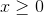: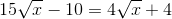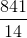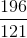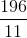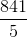Explanation:

Begin by getting yourterms onto the left side of the equation and your numeric values onto the right side of the equation: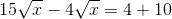Next, you can combine your radicals. You do this merely by subtracting their respective coefficients: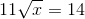Now, square both sides: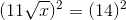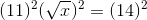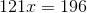Solve by dividing both sides by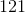: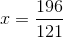### Example Question #3 : Square Roots And Operations

Simplify the following expression: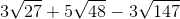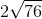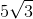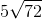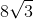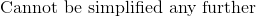Explanation:

To solve this problem, we must realize that the only way to add or subtract square roots is if the number under to square root is equivalent to each other. Therefore we must find a way to simplify each square root.

First we attempt to simplify the first term,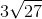We break apart the number under the square root and find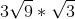Simplifying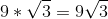Therefore we know that in order to try and simplify the other terms, the number under the square root has to be 3. By removing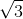from the other terms in the equation, we will attempt to see if they can be simplified as well.

For the second term,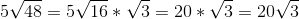Finally for the last term,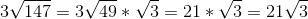Our new equation becomes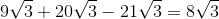Once all number under the square roots become the same, we can treat it as simple addition/subtraction and solve.